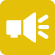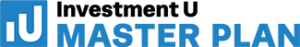## 1-Minute Survey

What Type of Investor Are You? – Take This 1-Min Survey to Find Out

Financial Literacy

# Simple Interest Calculator

Being able to calculate simple interest is key to your financial literacy. The simple interest calculator below will help determine the amount of interest charged on a loan, fixed deposit or savings account over a specific period of time. In other words, it is used to calculate monthly payments that are simple interest-only.

## How The Simple Interest Calculator Works

The simple interest calculator includes fields for:

• Loan Amount (in Dollars) – Put the amount borrowed into the area marked “loan amount.”
• Interest % – The “interest” field is where you should put the simple interest rate.
• Payment (Months or Years) – The result will appear as the minimum monthly payment based on the amounts that were input.

Clicking “Advanced Mode” will allow you to input the time frame in which the loan is taking place. It will also allow you to see the total interest amount in dollars.

• Time (Months of Years)
• Total Interest

Remember, simple interest does not take into account a reduction of the principal. With simple interest, the principal and the interest rate will remain the same during a specific period of time.

## Simple Interest Explained

In short, simple interest tells you the total cost of borrowing a set amount of money. As you can see, simple interest is very easy to determine using the simple interest calculator. Simple interest is most typically used for loans that last no longer than a year. However, there may be occasions where this is not the case. Once a loan crosses the threshold of 12 months, another concept called “compound interest” is introduced into the equation. For more information, check out our compound interest calculator.

When using the simple interest calculator, the formula Interest = Principle x rate x time period should be remembered. These variables are necessary in order to arrive at the correct amount.

Understanding the basic foundation of interest is key. Interest and its overall impact on your finances will help you on your path to financial freedom.

For the latest finance and investing news, signup for our free Investment U e-letter below.